# Maya summarySummary of elementary Maya feature.

# Shortcuts

### Camera

 alt + left click Rotate alt + middle click Strafe / pan alt + right click Zoom F Focus on selection

### Other

 S Insert key frame

# Architecture

Maya works on nodes. Nodes have attributes which we connect together using MEL or Python scripts.

Maya GUI calls those script to setup the nodes.

# MEL script

## Manual

Bookmark the MEL reference manual of whatever Maya version you are using. Once you get an understanding of MEL scripting this is the go to page to find all MEL built-in commands.

## Useful resources

MEL syntax (quite good and concise):

http://nccastaff.bournemouth.ac.uk/jmacey/OldWeb/RobTheBloke/www/

## Syntax

Variables are always prefixed with '$' when declared and used: int$myInt = 0;
string $helloString = "hello"; print($helloString + " " + $myInt ); // outputs: 'hello 0'  Declaring arrays: int$myArray[] = {1,3,9};


for loops (notice we don't declare the types):

for( $i=0,$j=0.0; $i<10; ++$i, $j+=0.75 ){ print($j + ", ");
}
// outputs: '0, 0.75, 1.5, 2.25, 3, 3.75, 4.5, 5.25, 6, 6.75,'


Iterate over array elements:

int $myArray[] = {1,3,9}; for($i = 0; $i < size($myArray); ++$i) print($myArray[$i] + ", "); // outputs: '1, 3, 9,' // Alternatively: for($element in $myArray) print($element + ", ");


MEL arrays are dynamic

int $myArray[] = {1,3,9};$myArray = -1;
for($i = 0;$i < size($myArray); ++$i)
print($myArray[$i] + ", ");
// outputs: 1, 3, 9, 0, 0, 0, 0, 0, 0, -1,


## Array /multi attributes

Maya nodes contain attributes (access through 'plugs' in c++/python API). When an attribute holds an array we also call it a multi attribute or array attribute.

Multi attributes can be thought as a map (e.g. map<unsigned (connection idx), Type (value)>):

     attribute (value) : [ elt1,  elt2,  elt3]
Logical indices (key): {    0,     2,     9} // indices displayed in 'Node Editor'
Physical indices     : {    0,     1,     2} // Always consecutive


Look up an attribute's connection and list the logical indices of the connection:

string $node_names[] = listConnections ($source_node+".attribute_name");
// example output: {'node1', 'node2'}

int $connection_indices[] = getAttr -multiIndices ($source_node+".attribute_name");
// example output: {0, 4}

// Loop through the nodes connected to the attribute '$source_node+".attribute_name[]"' for($i = 0; $i < size($node_names); ++$i) { int$idx = $connection_indices[$i];
string $name =$node_names[$i]; }  How to describe an attribute / plug: string$plug_name = "some_node_name.some_attr_name[index_atrr].some_compound_name"


Check connection at index:

Is a source {array} attribute currently connected to a node?
This can be use to determine if an index of an array attribute is free and can be safely connected with connectAttr().

string $con = connectionInfo -sourceFromDestination ("some_node_name.some_attribute[index]"); if( size($con ) == 0){
/* Yea I'm free */
}


Find first available connection (maya doc):

maya2014/scripts/AETemplates/getNextFreeMultiIndex.mel
source "getNextFreeMultiIndex.mel"
// N.B: no need to define index with [idx]
string $attribute_name = "some_node_name.some_attr_name"; // index from which we start looking up the array attribute. int$start_idx = 0;
getNextFreeMultiIndex(attribute_name, start_idx);


Remove element at index:

// This will remove the element with index 4 from the input of
// the choice node as long as there are no incoming or outgoing
// connections to the attribute.
removeMultiInstance choice.input;
// This will remove the element with index 100 from the input of
// the choice node, breaking any existing connections first.
removeMultiInstance -b true choice.input;


c.f maya2014/scripts/AETemplates/AEnewNonNumericMulti.mel and AEremoveMultiElement()

Get the size of the array attribute:

getAttr -size "some_node_name.some_attribute" // N.B: no need to define index with [idx]


List the currently used attribute:

listAttr -multi \$plug_name

Source of inspiration to understand how to handle multi-attributes:

maya2014/scripts/AETemplates/AEnewNonNumericMulti.mel

Check out functions like: AEnewNonNumericMultiAddNewItem(), AEremoveMultiElement()

## Mesh

Get number of vertices of a mesh:

polyEvaluate -v  "pCylinder1Shape";

# C++ API

## Introduction

Every data (DAG nodes, lights etc.) are represented as MObject in Maya. Instead of converting the MObject to some specialized type we use function sets MFnXxx(). To access the methods of a specialized type we must use a function set:

MFnAttribute attribute( mObject ); // bind object to functions
attribute.name(); // use object's functions
// We can also bind the function set like so:
attribute.setObject( mObject );


## Basics:

Display information in Maya script console: MGlobal::displayInfo("some infos");

Look up the DAG (scene tree), you can start from an arbitrary node with reset, use breadth or depth first order and check the current depth of the iterator (0 for root):

MItDag aItDag(MItDag::kDepthFirst);
aItDag.reset(iNode.mDagPath.node(), MItDag::kDepthFirst);
for(; !aItDag.isDone(); aItDag.next())
{
MFnDagNode fnNode( aDagPath ); // Get the DAG node
MString str = fnNode.typeName(); // Get its type for instance "transform" or "joint"
aItDag.depth();// Depth in the scene tree

}

Alternate way to look up the scene tree:

void rec_tree(const MFnDagNode& fnNode)
{
for(unsigned i = 0; i < fnNode.childCount(); ++i) {
MObject obj = fnNode.child( i );
ret_tree(obj); // Sometimes this conversion fails between MFnNode and MObject wherase the dag iterator works well TODO: search why.
}
return;
}
MFnDependencyNode fn( dagPath );
// get the MPlug for the tx attribute
MPlug plug = fn.findPlug("tx");
// get the value from the plug
float tx;
plug.getValue(tx);


Custom nodes: Construction: Never call Maya API in the custom node. Instead override the method: postConstructor() Destruction: When removing a node with deleteNode the key stroke del etc. you only remove the node from the dependency graph and the node is placed onto the undo list. The destructor will not be called unless the node disappear from the undo list (list flush/overflow, new scene) Removal from the dependency graph can be detected with various callbacks, see: MDGMessage:: MNodeMessage::addNodeAboutToDeleteCallback etc.

# Python API

Mostly resemble the C++ API. There are two python APIs:

#Old
from maya.OpenMaya import *
# Python API 2.0
from maya.api.OpenMaya import *


Be sure to not mix them up (especially when looking for documentation they are very similar) and use the latest one for new code.

## Tricks

Quick way to check environment variables (PATH, MAYA_PLUGIN_PATH etc)

import sys
for elt in sys.path:
print(elt)

import os, pprint
pprint.pprint(dict(os.environ), width = 1)


# Maya math nodes

plusMinusAverage math node specification. Originally designed with shaders in mind those operations work on vectors, i.e. addition, division etc is usually applied on a per component basis (out.x = in1.x + in2.x), (out.y = in1.y + in2.y) etc.

 Note: when disconnecting make sure to reset the value of the attribute, otherwise it will keep adding, subtracting etc. whatever was the value at disconnection. SUM:     Output1D = $$\sum_i$$ Input1D[ i ]     Output2Dx = $$\sum_i$$ Input2D[ i ]x     Output2Dy = $$\sum_i$$ Input2D[ i ]y     Output3Dx = $$\sum_i$$ Input3D[ i ]x     Output3Dy = $$\sum_i$$ Input3D[ i ]y     Output3Dz = $$\sum_i$$ Input3D[ i ]z SUBSTRACT:     Output1D = Input1D[ 0 ] - $$\sum_{i+1}$$ Input1D[ i ]     Output2Dx = Input2Dx[ 0 ] - $$\sum_{i+1}$$ Input2Dx[ i ]     Output2Dy = Input2Dy[ 0 ] - $$\sum_{i+1}$$ Input2Dy[ i ]     Output3Dx = Input2Dx[ 0 ] - $$\sum_{i+1}$$ Input2Dx[ i ]     Output3Dy = Input2Dy[ 0 ] - $$\sum_{i+1}$$ Input2Dy[ i ]     Output3Dz = Input2Dz[ 0 ] - $$\sum_{i+1}$$ Input2Dz[ i ] AVERAGE: Note: when disconnecting make sure to delete the array element otherwise it will average based on the current size.     N = getAttr -size plusMinusAverage.Input1D     Output1D = ( $$\sum_i$$ Input1D[ i ] ) / N     N = getAttr -size plusMinusAverage.Input2D     Output2Dx = ( $$\sum_i$$ Input2D[ i ]x ) / N     Output2Dy = ( $$\sum_i$$ Input2D[ i ]y ) / N     N = getAttr -size plusMinusAverage.Input3D     Output3Dx = ( $$\sum_i$$ Input3D[ i ]x ) / N     Output3Dy = ( $$\sum_i$$ Input3D[ i ]y ) / N     Output3Dz = ( $$\sum_i$$ Input3D[ i ]z ) / N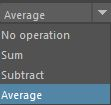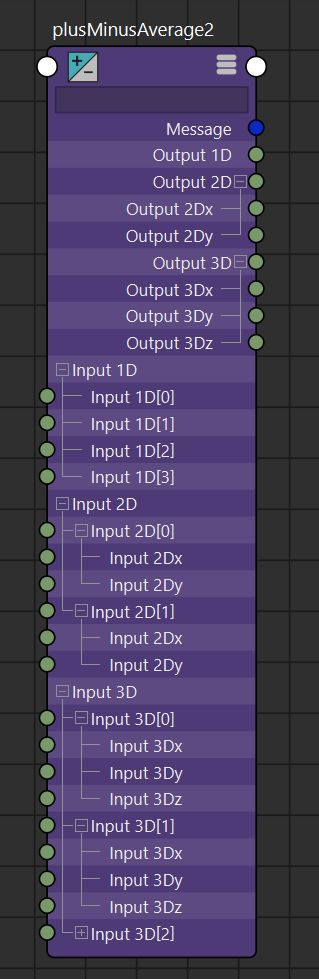divideMultiply math node specification.

 NO OPERATION:     Output.x = Input1.x     Output.y = Input1.y     Output.z = Input1.z     Input2.xyz is ignored. MULTIPLY:     Output.x = Input1.x * Input2.x     Output.y = Input1.y * Input2.y     Output.z = Input1.z * Input2.x DIVIDE:     Output.x = Input1.x / Input2.x     Output.y = Input1.y / Input2.y     Output.z = Input1.z / Input2.x POWER:     Output.x = $$(Input1.x) ^ { Input2.x }$$     Output.y = $$(Input1.y) ^ { Input2.y }$$     Output.z = $$(Input1.z) ^ { Input2.x }$$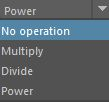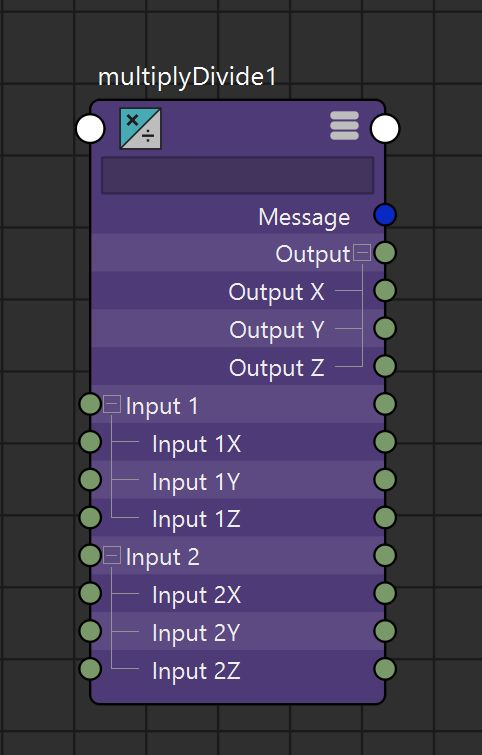condition math node specification. Compare 'firstTerm' and 'secondTerm' with some boolean operator (<,>,== etc.) OutColor is assigned ColorIfTrue or ColorIfFalse according to the result of the operation.

 if( firstTerm operation secondTerm ){     OutColor.r = ColorIfTrue.r     OutColor.g = ColorIfTrue.g     OutColor.b = ColorIfTrue.b } else {     OutColor.r = ColorIfFalse.r     OutColor.g = ColorIfFalse.g     OutColor.b = ColorIfFalse.b }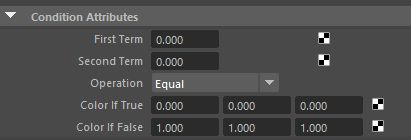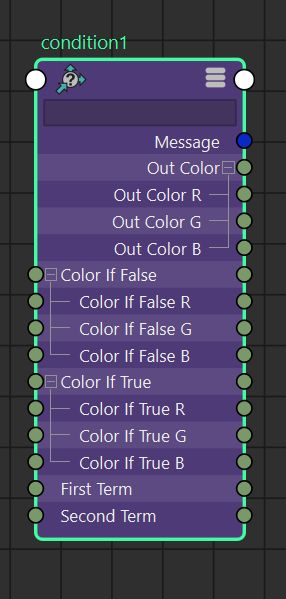BlendColors math node specification. Linear interpolation between Color1 and Color2 given the parameter $$blender \in [0.0, 1.0]$$.

 Output = Color1.rgb * (1.0 - blender) + Color2.rgb * blender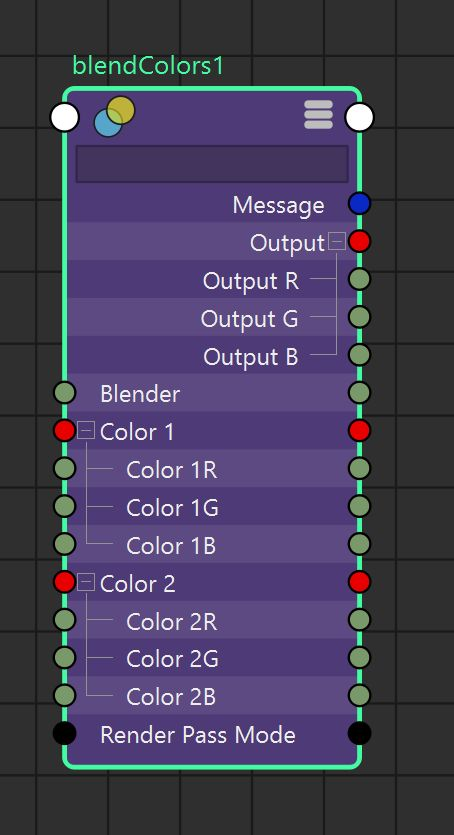Reverse math node specification. Reverse a parametric parameter: Output = 1.0 - Input.

 Output.x = 1.0 - Input.x     Output.y = 1.0 - Input.y     Output.z = 1.0 - Input.z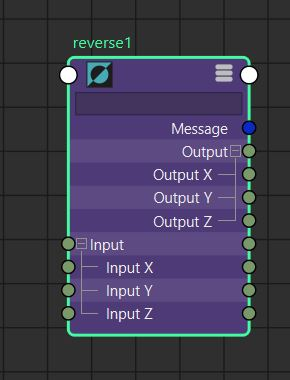Remaps an input scalar to an output scalar according to a user defined curve. Also outputs a color according to the position of the input scalar into the user defined color gradient.
Note: always prefer 'remapValue' which is really fast to evaluate contrary to the 'animCurve' node.
 OutputValue = Curve( InputValue ) Where the function "float Curve(float)" is the curve displayed in the attribute editor and which control points are stored in the "Value[]" attribute; OutputColor.RGB = Gradient( InputValue ) Where the function "Vec3 Gradient(float)" is the gradient strip displayed in the attribute editor and which interpolated colors are stored in the "Color[]" attribute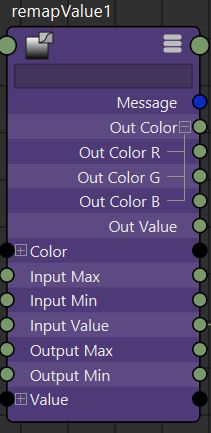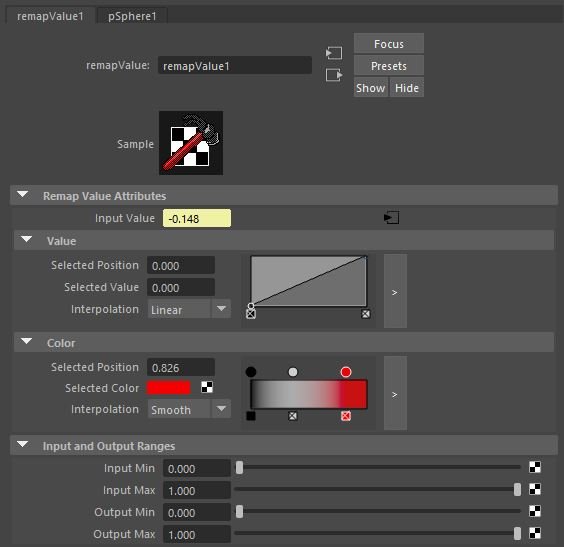## Matrices

Official list of built in matrix nodes

Multiplication order, from right to left as we increase the index:

Indices:     n      ...        1       0
Matrix :  mat_n  *  ...  *  mat_1 * mat_0


TODO: addMatrix, aimMatrix, blendMatrix, composeMatrix, decomposeMatrix, fourByFourMatrix, holdMatrix, passMatrix, pickMatrix, uvPin, proximityPin

Multiplies a list of 4x4 matrices together.
 $$MatrixSum = \prod_{i=n}^{0} MatrixIn[i]$$ MatrixSum = MatrixIn[n] * ... * MatrixIn * MatrixIn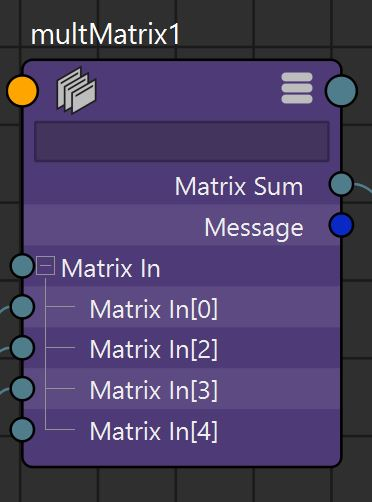Weighted sum of a list of 4x4 matrices.
 $$MatrixSum = \sum_{i=n}^{0} WtMatrix[i].MatrixIn * WtMatrix[i].WeightIn$$ MatrixSum = WtMatrix[n].MatrixIn * WtMatrix[n].WeightIn * ... * WtMatrix.MatrixIn * WtMatrix.WeightIn * WtMatrix.MatrixIn * WtMatrix.WeightInExtra nodes in 'matrixNodes.mll' plugin:
- inverseMatrix node
- transposeMatrix node